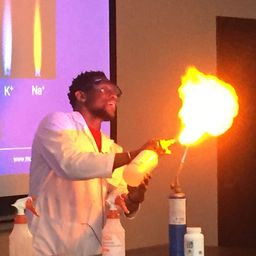### Calculate the molar mass of each of the following…

ViewProblem 11

How are the molecular mass and the molar mass of a compound similar and how are they different?

Both molecular mass and molar mass have the same numerical value, however,
they have different units.
Molecular mass is in amu or atomic mass units and it represents the mass
one 1 molecule.
Molar mass is in grams and it represents the mass of 1 mole of molecules
$\left(6.022 \times 10^{23} \text { molecules). }\right.$

## Discussion

You must be signed in to discuss.

## Video Transcript

in this question. We've been asked to explain how the medical mass and Mullah massive compound differ. And how are they similar? So the first name to notice that the mobile mast in the mullahs mus give us the same Miracle Valley. So what I'm trying to say is that the mocha Massimo Lemus off a compound or let's say an element, for instance, it's the same. Where is his oxygen? Right? Malcolm asking the mullahs mass have the signal. Recall valley for oxygen. The molecular mass is 15.999 in the mullahs Mass is also 15.9 I. Where did do differ is in the units, right? So the differing the units. So the units off the mocha masses usually atomic mass units given by simple am you in the units for the mullahs Masses grams more so there. Welcome. Ass is a massive one molecule. And then the mullahs mask the massive one mole off the mall.